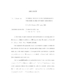## Universal bounds on coarsening rates for some models of phase transitions##### Files
umi-umd-2235.pdf(410.6 KB)
In this thesis, we prove one-sided, universal bounds on coarsening rates for three models of phase transitions by following a strategy developed by Kohn and Otto (Comm. Math. Phys. 229(2002),375-395). Our analysis for the phase-field model is performed in a regime in which the ratio between the transition layer thickness and the length scale of the pattern is small, and is also small compared to the square of the ratio between the pattern scale and the system size. The analysis extends the Kohn-Otto method to deal with both temperature and phase fields. For the mean-field models, we consider two kinds of them: one with a coarsening rate $l\sim t^{1/3}$ and the other with $l\sim t^{1/2}$. The $l\sim t^{1/2}$ rate is proved using a new dissipation relation which extends the Kohn-Otto method. In both cases, the dissipation relations are subtle and their proofs are based on a residual lemma (Lagrange identity) for the Cauchy-Schwarz inequality. The monopole approximation is a simplification of the Mullins-Sekerka model in the case when all particles are non-overlapping spheres and the centers of the particles do not move. We derive the monopole approximation and prove its well-posedness by considering a gradient flow restricted on collections of finitely many non-overlapping spheres. After that, we prove one-sided universal bounds on the coarsening rate for the monopole approximation.